Get instant live expert help with Excel or Google Sheets“My Excelchat expert helped me in less than 20 minutes, saving me what would have been 5 hours of work!”

#### Post your problem and you’ll get Expert help in seconds.

Your message must be at least 40 characters
Our professional Expert are available now. Your privacy is guaranteed.

# How to Count Cells with Over 100 CharactersRead time: 20 minutes

We can use a formula that combines the SUMPRODUCT, N and LEN functions to count cells with over 100 characters. The steps below will walk through the simple process.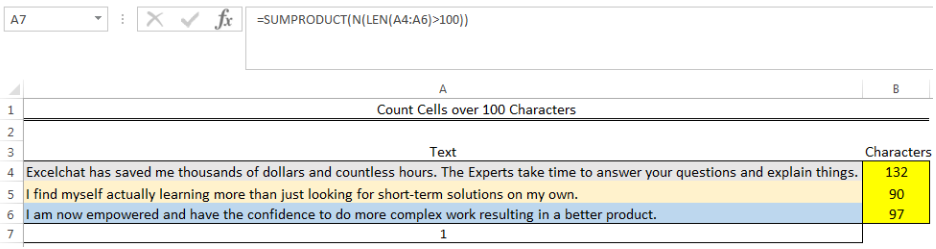Figure 1: Resulting showing that only one cell has characters over 100

## General Formula

`=SUMPRODUCT(N(LEN(array)>100))`

## Formula

`=SUMPRODUCT(N(LEN(A4:A6)>100))`

## Setting up the Data

We will count the number of cells with over 100 characters using the text in Cell A4 to Cell A6 as shown in figure 2.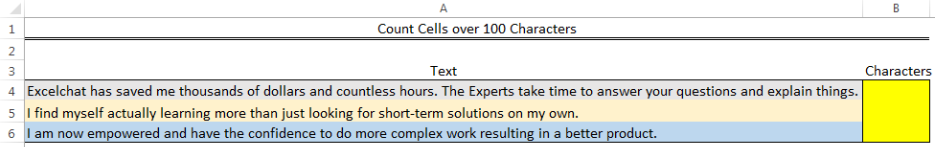Figure 2: Setting up the Data

## Getting the Characters

• To get the characters, we will input the formula below into Cell B4

`=LEN(A4)`

• We will press the enter key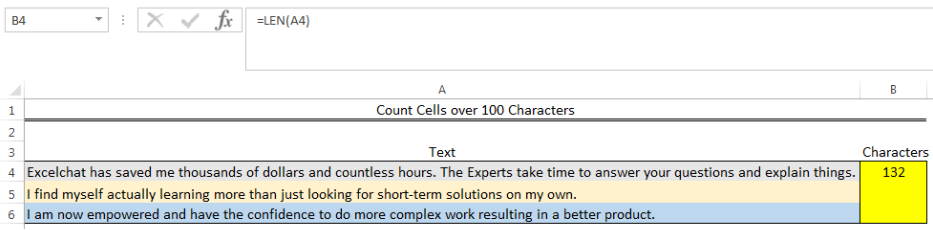Figure 3: Getting the Characters

• We will click on Cell B4 again
• We will double-click on the fill handle (the small plus sign at the bottom right of Cell B4) and drag down to copy the formula into the other cells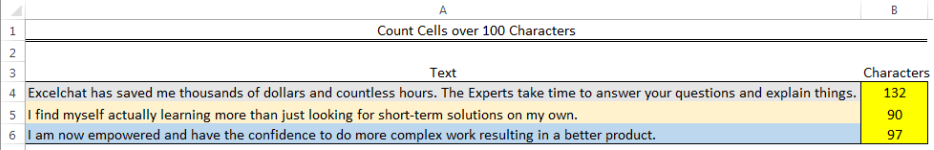Figure 4: Getting the Characters

## Counting Cells Over 100 Characters

• We will click on Cell A7 and insert the formula below

`=SUMPRODUCT(N(LEN(A4:A6)>100))`

• We will press the enter key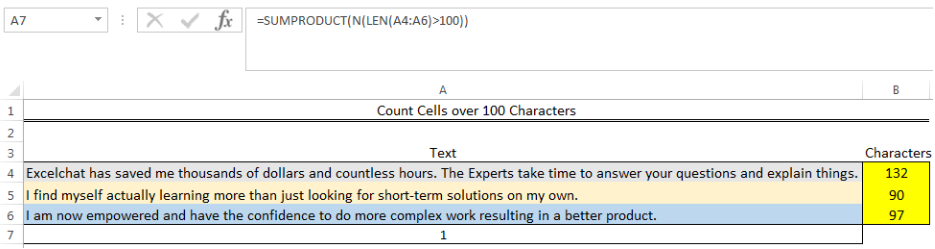Figure 5: Resulting showing that only one cell has characters over 100

## Explanation

The LEN function returns the number of characters in each cell within the range (A4:A6) as an array. The result looks like this: `{132;90;97}`

The returned array result is then checked with the logical expression if the values are greater than 100. This returns an array of TRUE and FALSE values: `{TRUE;FALSE;FALSE}`

The N function converts the returned values to ones and zeroes: `{1;0;0}`

The SUMPRODUCT function finally sums the returned array result, which returns 1.

## Instant Connection to an Expert through our Excelchat Service

Most of the time, the problem you will need to solve will be more complex than a simple application of a formula or function. If you want to save hours of research and frustration, try our live Excelchat service! Our Excel Experts are available 24/7 to answer any Excel question you may have. We guarantee a connection within 30 seconds and a customized solution within 20 minutes.

### Did this post not answer your question? Get a solution from connecting with the expert.Another blog reader asked this question today on Excelchat:
Solution exampleshow to make a format that if multiple cells have number contents its value is 1 in the last cell
Solved by C. C. in 28 minsI need to find out if the 2018 yearly sales goals were met if the yearly sales were \$25,000 or more using an IF logical function and to set the formula to return a value of YES if met, and NO if not
Solved by A. A. in 18 minsI'm looking for a formula or format for if there is data in c4 on a sheet... It adds extra information underneath
Solved by X. E. in 20 minsï?·In cell G1enter Discount, ï?·In cell H1enter DiscountPercent,ï?·In cell I1enter DiscountCost,ï?·In cell J1enter TotalCost, andï?·In cell K1enter Paymentas column headings.
Solved by K. E. in 40 minsHi, I have a problem with vlookup formula, I am not sure what am I doing wrong but it seems to not be working
Solved by K. F. in 40 mins## Subscribe to Excelchat.coAnother blog reader asked this question today on Excelchat: